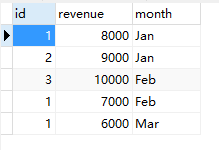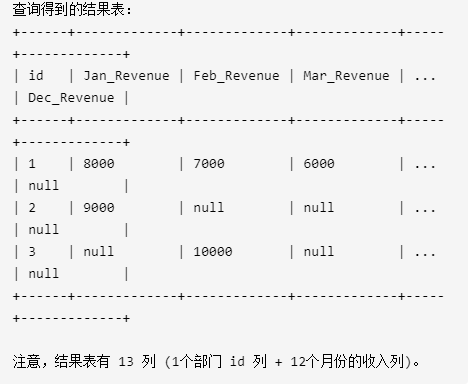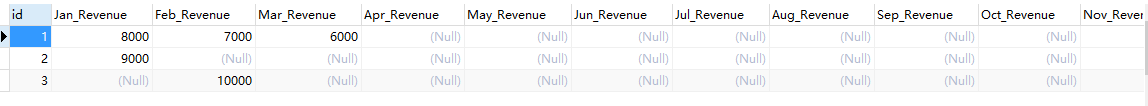# 行转列select
id
, sum(case month when 'Jan' then revenue else null end) as Jan_Revenue
, sum(case month when 'Feb' then revenue else null end) as Feb_Revenue
, sum(case month when 'Mar' then revenue else null end) as Mar_Revenue
, sum(case month when 'Apr' then revenue else null end) as Apr_Revenue
, sum(case month when 'May' then revenue else null end) as May_Revenue
, sum(case month when 'Jun' then revenue else null end) as Jun_Revenue
, sum(case month when 'Jul' then revenue else null end) as Jul_Revenue
, sum(case month when 'Aug' then revenue else null end) as Aug_Revenue
, sum(case month when 'Sep' then revenue else null end) as Sep_Revenue
, sum(case month when 'Oct' then revenue else null end) as Oct_Revenue
, sum(case month when 'Nov' then revenue else null end) as Nov_Revenue
, sum(case month when 'Dec' then revenue else null end) as Dec_Revenue
from Department group by id
select id,
sum(case when month = 'Jan' then revenue end) 'Jan_revenue',
sum(case when month = 'Feb' then revenue end) 'Feb_revenue',
sum(case when month = 'Mar' then revenue end) 'Mar_revenue',
sum(case when month = 'Apr' then revenue end) 'Apr_revenue',
sum(case when month = 'May' then revenue end) 'May_revenue',
sum(case when month = 'Jun' then revenue end) 'Jun_revenue',
sum(case when month = 'Jul' then revenue end) 'Jul_revenue',
sum(case when month = 'Aug' then revenue end) 'Aug_revenue',
sum(case when month = 'Sep' then revenue end) 'Sep_revenue',
sum(case when month = 'Oct' then revenue end) 'Oct_revenue',
sum(case when month = 'Nov' then revenue end) 'Nov_revenue',
sum(case when month = 'Dec' then revenue end) 'Dec_revenue'
from Department
group by id

select
id,
max(if(month = 'Jan',revenue,null)) Jan_Revenue,
max(if(month = 'Feb',revenue,null)) Feb_Revenue,
max(if(month = 'Mar',revenue,null)) Mar_Revenue,
max(if(month = 'Apr',revenue,null)) Apr_Revenue,
max(if(month = 'May',revenue,null)) May_Revenue,
max(if(month = 'Jun',revenue,null)) Jun_Revenue,
max(if(month = 'Jul',revenue,null)) Jul_Revenue,
max(if(month = 'Aug',revenue,null)) Aug_Revenue,
max(if(month = 'Sep',revenue,null)) Sep_Revenue,
max(if(month = 'Oct',revenue,null)) Oct_Revenue,
max(if(month = 'Nov',revenue,null)) Nov_Revenue,
max(if(month = 'Dec',revenue,null)) Dec_Revenue
from Department group by id01-2909-0910万+
04-133700
10-021万+
08-2310万+
04-023万+
11-142407
06-036万+